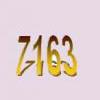#### You may also like### Old Nuts

In turn 4 people throw away three nuts from a pile and hide a quarter of the remainder finally leaving a multiple of 4 nuts. How many nuts were at the start?### Some Cubes

The sum of the cubes of two numbers is 7163. What are these numbers?### Em'power'ed

Find the smallest numbers a, b, and c such that: a^2 = 2b^3 = 3c^5 What can you say about other solutions to this problem?

# Factorial Fun

##### Age 16 to 18Challenge Level

We denote the product of the first 20 natural numbers by 20! and call this 20 factorial.

(a) What is the highest power of 5 which is a divisor of 20 factorial? Just how many factors does 20! have altogether?

(b) Show that the highest power of $p$ that divides $500!$, where $p$ is a prime number and $p^t < 500 < p^{t+1}$, is $$\lfloor 500/p\rfloor+\lfloor 500/p^2\rfloor+\dotsb+\lfloor 500/p^t\rfloor,$$ where $\lfloor x\rfloor$ (the floor of $x$) means to round down to the nearest integer.  (For example, $\lfloor 3\rfloor=3$, $\lfloor 4.7\rfloor=4$, $\lfloor -2.7\rfloor=-3$, and so on.)

(c) How many factors does $n!$ have?